# Lecture 13: Drawing Binary Trees I

## Annoucements

• Assignment 06 due tonight
• Assignment 07 posted soon
• make a site that incorporates recursion and coordinate transformation to make a self-similar image
• due next Monday
• Quiz this Wednesday: coordinate transformations
• define a matrix transformation given transformation’s geometric description
• given a matrix and original image, draw the transformed image

## Outline

1. Binary Trees
2. Activity: Drawing Binary Trees by Hand
3. Aesthetic and Pragmatic Principles
4. Greedy Procedure
5. Knuth Layout

## A While Back

We illustrated the depth-first search algorithm on graphs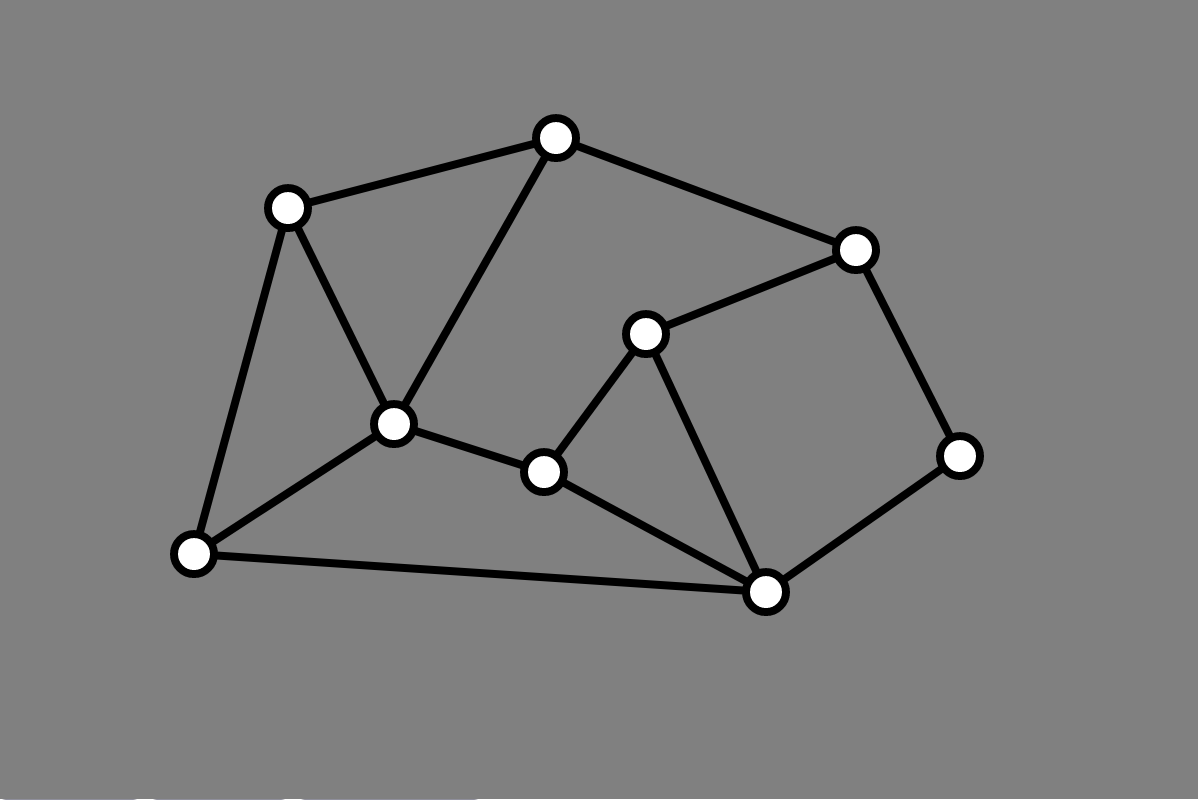## A While Back

We illustrated the depth-first search algorithm on graphs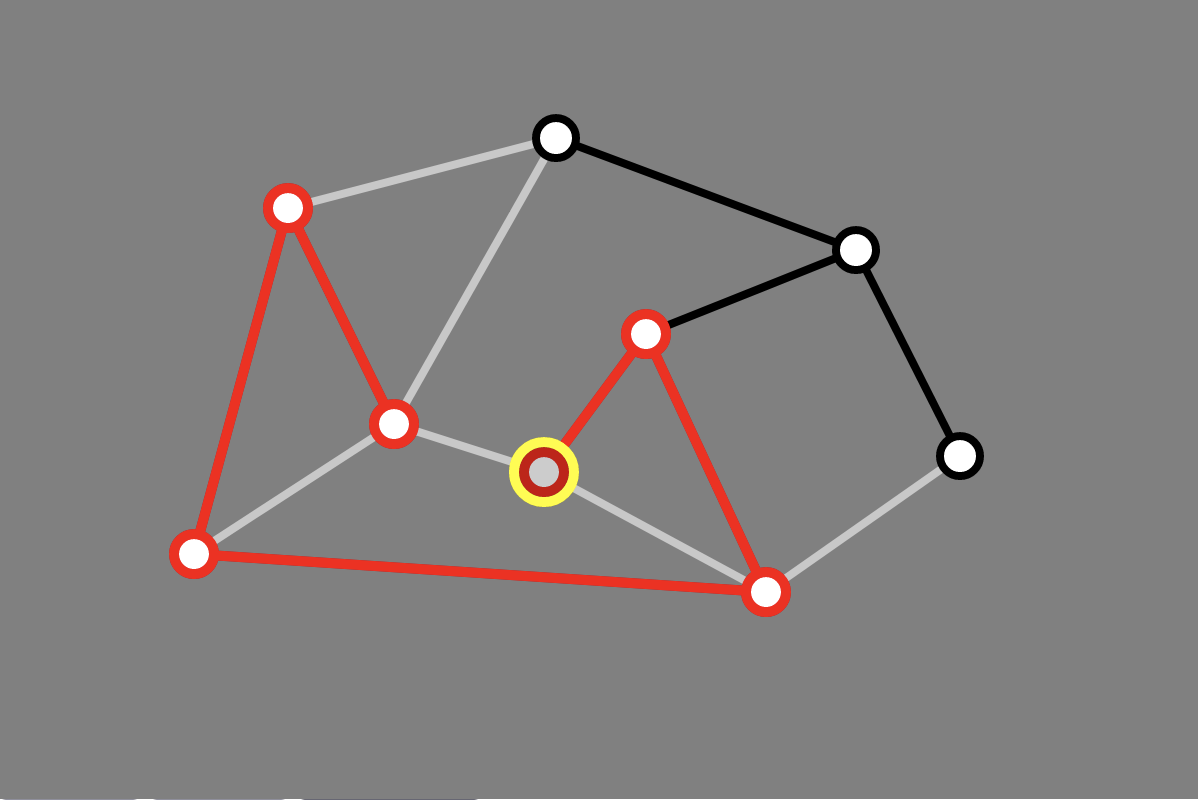## Interaction

User drew input graph graph by hand

• from clicks, obtained graph structure
• geometry of graph layout defined with graph

## Graph Drawing

Input. A graph

• vertices
• edges

Output. A drawing of the graph

• visual representation of vertices
• geometric locations
• usually “points”
• visual representation of edges
• usual lines or curves between vertices

## This Week

Algorithms for drawing binary trees

## Recall

A (rooted) binary tree consists of

• a set $V$ of vertices
• a root vertex
• each vertex has:
• a left child (possibly null)
• a right child (possibly null)

satisfying:

• the root is not anyone’s child
• every node is the child of exaclty one node
• every node is a descendant of the root

## Example

• V = {0, 1, 2, 3, 4}
• root = 0
• left(0) = 2, right(0) = 3
• left(3) = 4, right(3) = 1
• unassigned children are null

## Activity: Draw a Tree

V = {0,...,13}
root: 0
left(0) = 1, right(0) = 2
left(1) = 3, right(1) = 4
right(2) = 5
left(4) = 6, right(4) = 7
right(5) = 8
left(8) = 9
left(9) = 10,  right(9) = 11
left(10) = 12, right(10) = 13


## You Drew This, Right?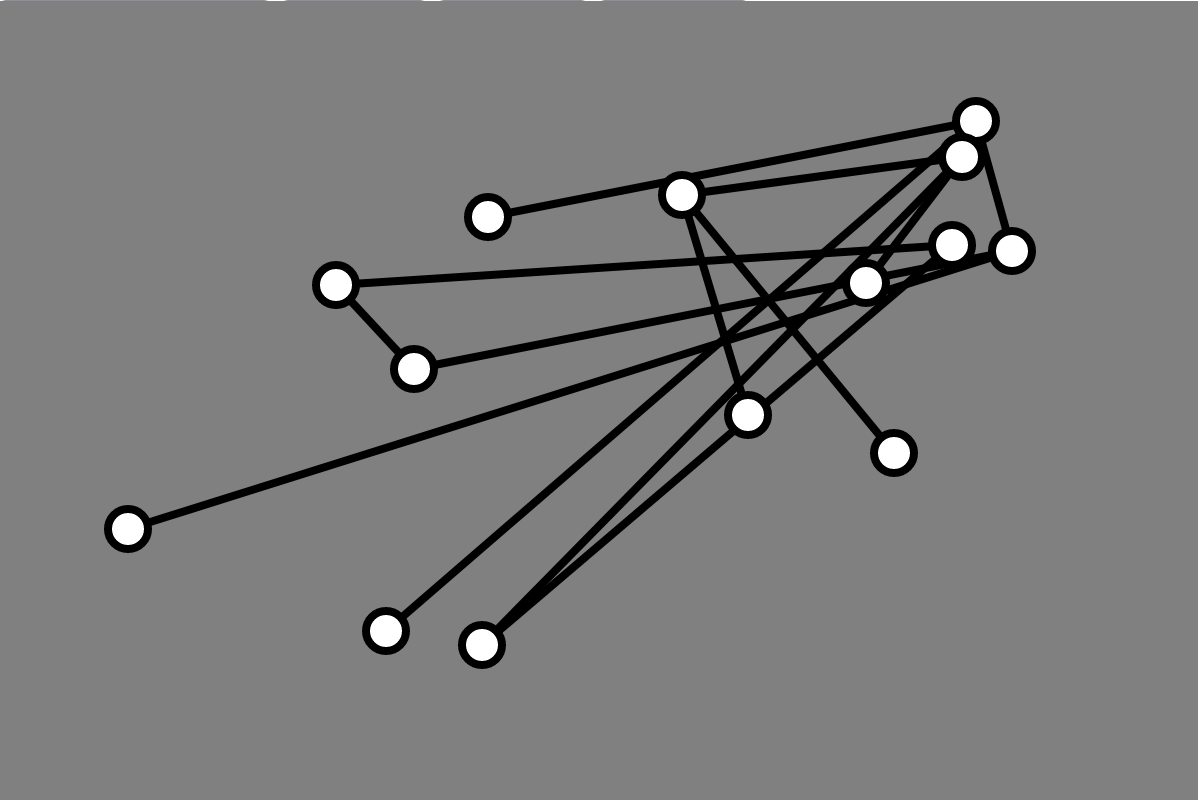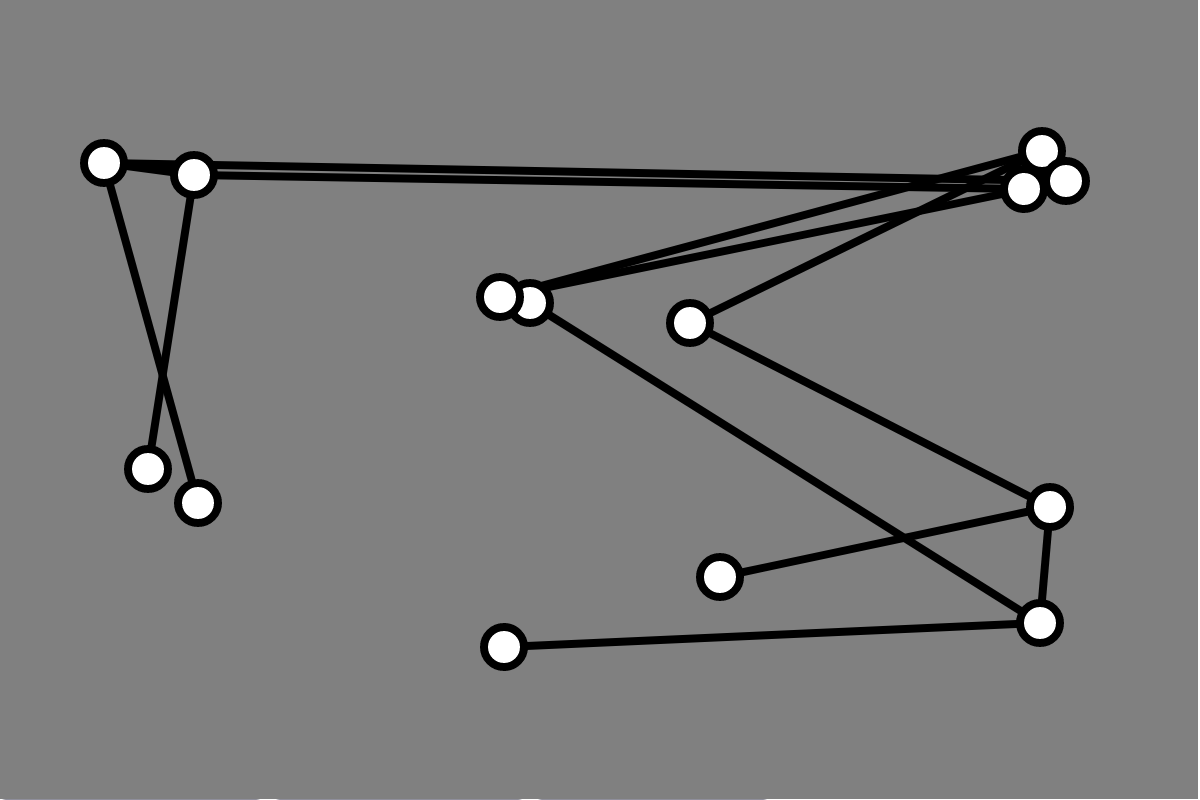## Questions

1. What information do we want to convey about the tree?
2. What constraints might we have on our drawing?
3. What aesthetic considerations might we have?
• when does a tree “look nice?”

## First Principle

Aesthetic Principle 1. Vertices at the same depth should lie along a horizontal line with deeper nodes lower than shallower nodes.

• what physical requirements does this impose?

## Physical Limitation

Have to contend with width

• What can we do about it?

## Optimal Layout?

How can we achieve minimum possible width subject to

1. lower bound on horizontal spacing
2. Aesthetic Principle 1

## Greedy Layout

Idea

• draw vertices in rows according to depth
• depth = distance from root
• root goes alone in the top row, next row at depth 1, etc.
• draw each row with vertices from “left to right”
• what does this mean?

Promise

• Use as few columns as possible!
• minimize width requirement

## Greedy Layout Illustrated

V = {0,...,7}
root: 0
left(0) = 1, right(0) = 2
left(1) = 3, right(1) = 4
right(2) = 5
left(4) = 6, right(4) = 7


## How to Implement Greedy Layout?

Input: tree (just the root?)

Output: row and column for each node

## How to Get Depths of Nodes?

My implementation:

• set depth when each vertex is added
• depth of a vertex is parent’s depth + 1
• store a Map:
• keys are vertex IDs
• values are depths

## How to Get Columns?

Observation. If $u$ is a left child of $v$ and $w$ is a right child of $v$, then $u$ should be in a column to the left of $v$.

Idea. Starting from the root:

1. place vertex in the left-most un-assigned column in its row (depth)
2. place left descendants
3. place right descendants

This is pre-order traversal!

## Column Assignment Illustrated

V = {0,...,7}
root: 0
left(0) = 1, right(0) = 2
left(1) = 3, right(1) = 4
right(2) = 5
left(4) = 6, right(4) = 7


## Greedy Layout in JavaScript

Computing Depths:

const BinaryTree = function (root) {
this.depths = new Map();
...
this.addLeftChild = function (parentID, childID) {
...
this.depths.set(childID, this.depths.get(parentID) + 1);
}
}


## Greedy Layout in JavaScript

Getting vertices in “pre-order”

this.verticesPreOrder = function (from = this.root) {
let vertices = [];
vertices.push(from);
if (from.left != null)
vertices = vertices.concat(this.verticesPreOrder(from.left));
if (from.right != null)
vertices = vertices.concat(this.verticesPreOrder(from.right));
return vertices;


## Greedy Layout in JavaScript

Getting Rows and Columns

this.setLayoutGreedy = function () {
const vertices = this.tree.verticesPreOrder();
const depths = this.tree.depths;
...
const cols = []; // current col for each row, initialized to 0
...
for (let vtx of vertices) {
let row = depths.get(vtx.id)
let col = cols[row];
cols[row]++;
/* set position of vtx to this row and col */
}}


## Demo

• lec13-binary-tree.zip

## What is Missing?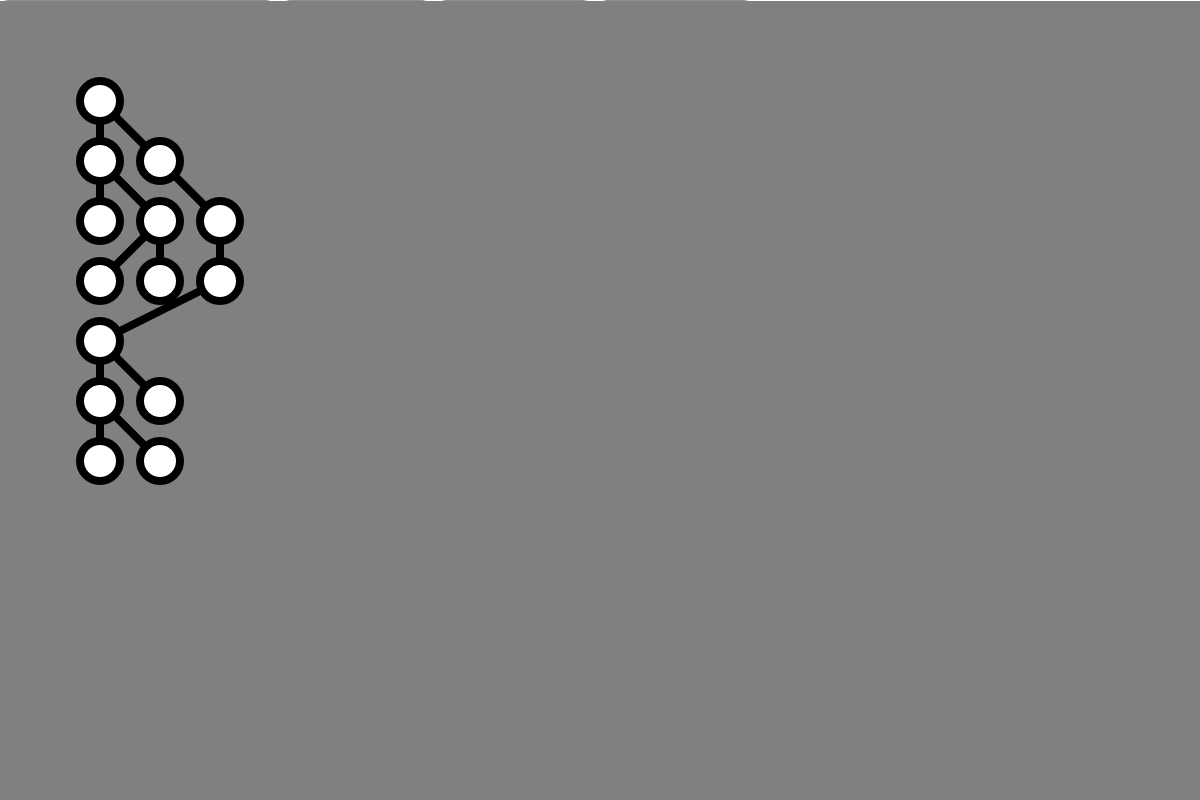## Second Principle

Aesthetic Principle 2. The left child of any node should appear to the left of its parent, and a right child should appear to the right of its parent.

## Knuth’s Layout Algorithm

Rows and Columns

• rows are defined by depth (Aesthetic Principle 1)
• columns are “in-order” traversal order
• each vertex gets own column
• guarantees
• left descendants to the left
• right descenadants to the right

## In-order Traversal in Code

this.verticesInOrder = function (from = this.root) {
let vertices = [];
if (from.left != null)
vertices = vertices.concat(this.verticesPreOrder(from.left));
vertices.push(from);
if (from.right != null)
vertices = vertices.concat(this.verticesPreOrder(from.right));
return vertices;


## Knuth’s Layout in Code

this.setLayoutKnuth = function () {
const vertices = this.tree.verticesInOrder();
const depths = this.tree.depths;
for (let i = 0; i < vertices.length; i++) {
let vtx = vertices[i];
let depth = depths.get(vtx.id);
/* set vtx location to row depth, column i */
}
}


## Result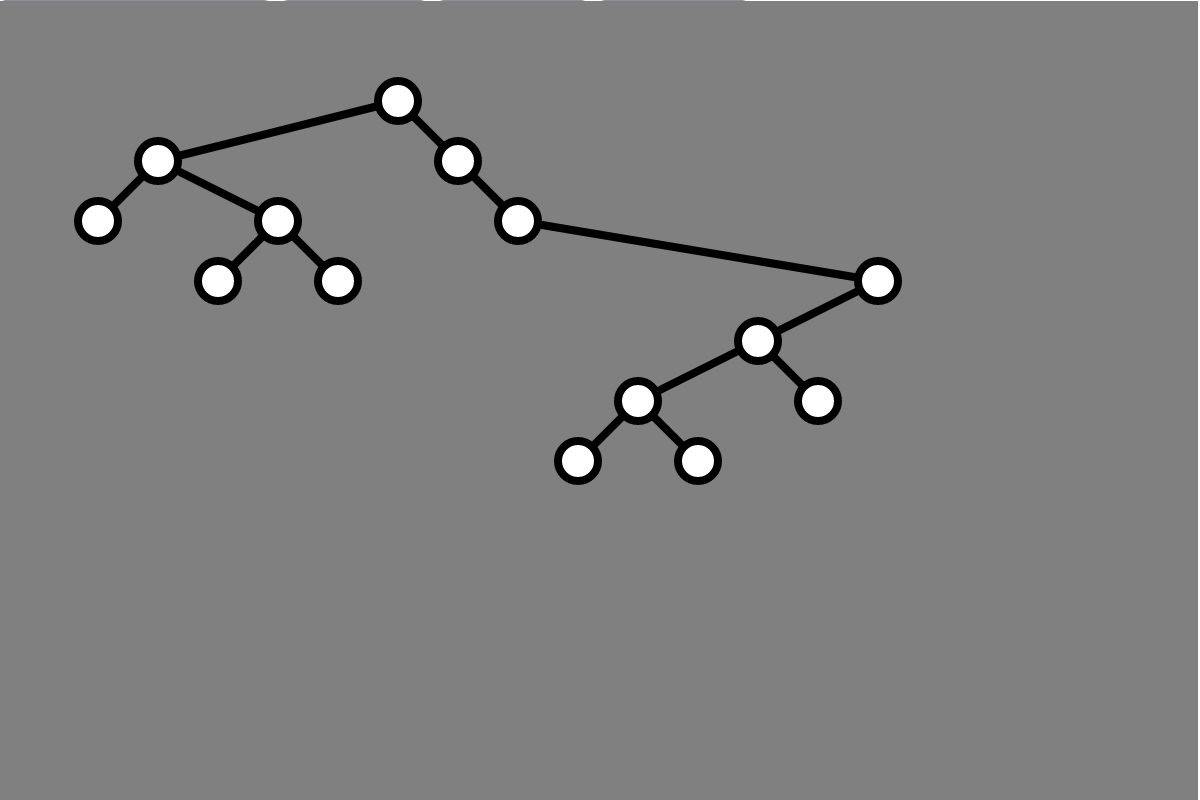## Demo, Again

• lec13-binary-tree.zip

## Result Again## Third Principle

Aesthetic Principle 3. If a node has two children, it’s $x$-coordinate should be the midpoint of its childrens’ $x$-coordinates

## Questions (Next Time)

1. How can we satisfy all three aesthetic principles?
2. How can all be satisfied while also minimizing the width of the drawing?
3. What tradeoffs are we forced to make balancinng these principles?